Objects and Methods : Aquifer : Dispatch Methods
Dispatch Methods
This object solves for Storage and Head.Solve given Head Previous
The Solve given Head Previous dispatch method computes the current Storage and Head values based on the mass balance equation described below.
The dispatch method execute when the Flow from Above is known and the Head Upstream/Downstream/Right/Left Previous slots are known. Depending on the number of connected objects, one or all of the Head Upstream/Downstream/Right/Left Previous slots may be active.
Required Known Slots
• Flow from Above, if using Specify or Link Flow in the Flow from Above category
• Head Above Previous, if using Compute Flow using Head in the Flow from Above category
• If using Compute Flow using Head in the respective Flow from L/R/U/D/B category:
Required Unknown Slots
Solution Equations
The dispatch method can be executed at each run timestep, but the method will exit unsuccessfully for any non-computational timestep. Following are the steps in the solution, followed by an example.
First, if the Input or Linked method is selected for Pumping, the Pumped Flow is aggregated into an average value over the computational timestep. If there are any values missing for the computational period, the method exits and waits for all values to be known.
The following equations are used when Compute Flow using Head is selected. This typically occurs when the aquifer is only connected to other Aquifer objects as shown in Figure 5.2. In this case, t is the computational timestep:
(5.14)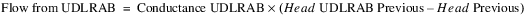(5.15)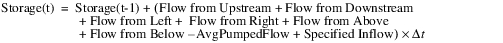Figure 5.2  Cross section of an Aquifer object with an Aquifer above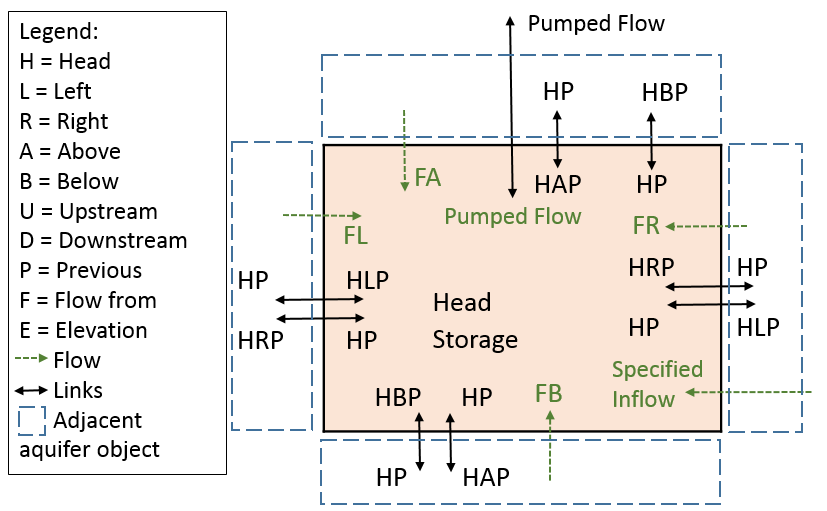Note:  Links and flows are not shown for Upstream and Downstream but they would follow the same convention.
The following equations are used when Specify or Link Flow is selected in the Flows from Above category. In this case, the aquifer typically has a Groundwater object above it. The variable t is the current computational timestep:
(5.16)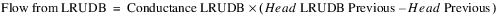(5.17)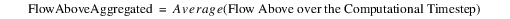(5.18)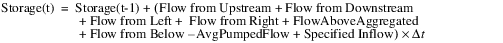Figure 5.3 shows this setup:
Figure 5.3  Cross section of an Aquifer object with Aquifer aboveFor both solution approaches, once the Storage is computed, the Head at the computational timestep is:
(5.19)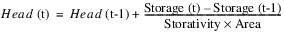Timing of the Solution
The following is an example that shows the timing and interaction of this solution with the run timestep and computational timestep. In this example, the run timestep is 1 day, while the computational timestep is 1 month. Figure 5.4 shows a diagram for the first four steps.
1. During run initialization, the Aquifer will be required to have Head and Storage at the initial timestep. On other timesteps, the Aquifer objects will dispatch at the end of each month and compute the aquifer storage and head for that month (as per step 9 below)
2. This head will be then set on a daily timestep slot called Head Previous for each day in the next month.
3. This slot, Head Previous, is linked to the shallow Groundwater object’s Deep Aquifer Elevation Previous and the values are propagated to it for each timestep in the next month.
4. When the shallow groundwater is able to solve at the run timestep, it will have the Deep Aquifer Elevation Previous and can solve for Percolation. The solution can then proceed for the Groundwater object through the month at a daily timestep. The Aquifer may dispatch, but will not do anything at a daily timestep.
5. On the second to last day of the month; that is, day 30, the shallow Groundwater object will compute an Elevation on day 30 and set the Elevation Previous on day 31. The Groundwater Elevation Previous is linked to the Aquifer’s Head Above Previous. This will trigger the Aquifer to dispatch.
6. Given the Head Previous (computed in step 2) and adjacent Head LRUDB Previous values, the Aquifer can compute the flows into the aquifer (Flow from LRUDB). Also, if the Link Heads is selected in the Flows Above category, calculate Flow from Above similarly.
7. If using the Specify or Link Flow method, the groundwater Percolation slot will propagate to the Flow from Above.
8. Given Flow from LRUDAB and any specified Pumped Flow and Specified Inflows, compute the Storage and Head for that month.
9. Go to step 2 and repeat.
Figure 5.4  Sample interaction of the Aquifer with a Groundwater object above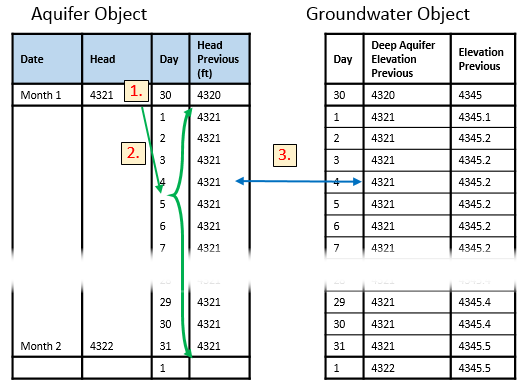Revised: 08/02/2021